参数 P02 = 0 P02 = 1 P02 = 2 P02 = 3 P02 = 4 序列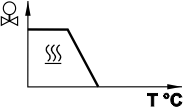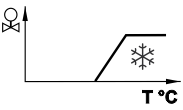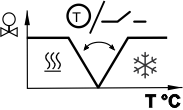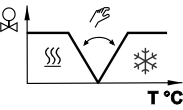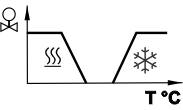可用的基本应用 ⇓ 仅供热 仅制冷 通过外部水温传感器或远程开关实现供热制冷自动转换 手动选择供热或制冷序列（通过 HMI） 供热和制冷序列，四管制 两管制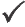四管制1)1) 关于四管制应用的手动转换，参见 四管制风机盘管

• 四管制手动转换 (P02 = 3) 指仅启用制冷或供热输出